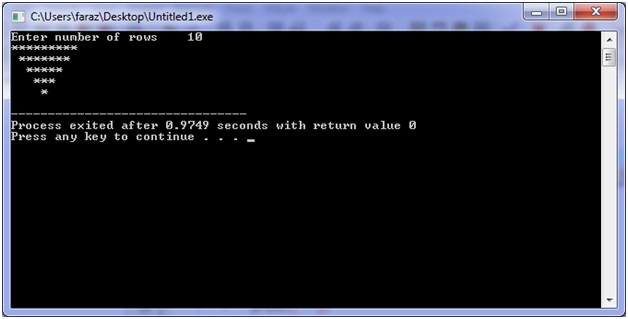# C program for 'Reverse Full Pyramid' Pattern

In this C program, we are implementing logic to print Reverse Full Pyramid pattern using asterisks and loops (nested loops).
Submitted by Shamikh Faraz, on February 16, 2018

In this program, we will learn, how we can print reverse full pyramid using ‘asterisks’ and ‘loops’.

C program

```#include <stdio.h>

int main()
{
int a, b, rows, space;

printf("Enter number of rows: ");
scanf("%d", &rows);
for (a = 5; a >= 1; a--) //decease number of rows
{
//print magin space
for (b = 1; b <= space; b++)
printf(" ");

//this loop prints half part of pyramid
for (b = 1; b <= a; b++)
printf("*");

//this loop prints remaining half part of pyramid
for (b = a - 1; b >= 1; b--)
printf("*");

printf("\n");
space++;
}

return 0;
}
```

Output:

```Enter number of rows: 5
*********
*******
*****
***
*
```